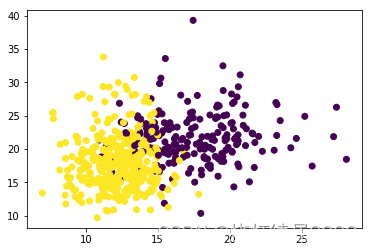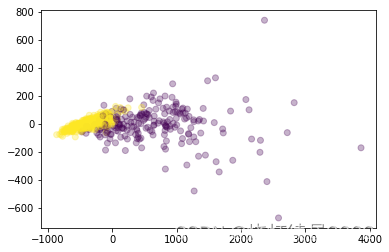# 支持向量机 - 探索核函数的优势和缺陷

• 2022-11-25
山东
• 本文字数：3253 字

阅读完需：约 11 分钟

from sklearn.datasets import load_breast_cancerfrom sklearn.svm import SVCfrom sklearn.model_selection import train_test_splitimport matplotlib.pyplot as pltimport numpy as npfrom time import timeimport datetime # 将时间戳转化为真实地时间data = load_breast_cancer()X = data.datay = data.targetX.shape---(569, 30)np.unique(y)---array([0, 1])

plt.scatter(X[:,0],X[:,1],c=y)
plt.show()from sklearn.decomposition import PCA

X_dr = PCA(2).fit_transform(X)
plt.scatter(X_dr[:,0],X_dr[:,1],c=y,alpha=0.3)
plt.show()Xtrain,Xtest,Ytrain,Ytest = train_test_split(X,y,test_size=0.3,random_state=420)Kernel = ["linear","poly","rbf","sigmoid"]# 注意以下这一段跑不出结果for kernel in Kernel:    time0 = time()    clf = SVC(kernel=kernel             ,gamma="auto"            # ,degree=1 # degree：核函数的次方数，默认是3              # 之前说过当degree为1的时候用于线性，高次用于非线性              # 但是之前使用默认值的时候跑了将近十分钟没有跑出poly的结果，放弃了             ,cache_size=5000             ).fit(Xtrain,Ytrain)    # cache_size：表示允许使用多大的内存进行计算，单位MB。默认200    # 用的内存越多运行越快（蓝屏可能性越大）    print("The accuracy under kernel %s is %f"%(kernel, clf.score(Xtest,Ytest)))    print(datetime.datetime.fromtimestamp(time()-time0).strftime("%M:%S:%f"))# 一般SVM不需要过长的时间，超过五六分钟不出结果基本就可以选择打断了---The accuracy under kernel linear is 0.92982500:00:396585

now = time() # 返回一串浮点数，就是时间戳

datetime.datetime.fromtimestamp(now).strftime("%Y-%m-%d,%H:%M:%S:%f")
---
'2022-10-24,10:33:16:370524'


Kernel = ["linear","rbf","sigmoid"] # 谁慢把谁删了for kernel in Kernel:    time0 = time()    clf = SVC(kernel=kernel             ,gamma="auto"            # ,degree=1              ,cache_size=5000             ).fit(Xtrain,Ytrain)    print("The accuracy under kernel %s is %f"%(kernel, clf.score(Xtest,Ytest)))    print(datetime.datetime.fromtimestamp(time()-time0).strftime("%M:%S:%f"))    # 基本秒出，所以证明是多项式核函数阻碍了我们的运行---The accuracy under kernel linear is 0.92982500:00:405324The accuracy under kernel rbf is 0.59649100:00:038619The accuracy under kernel sigmoid is 0.59649100:00:006005

Kernel = ["linear","poly","rbf","sigmoid"]for kernel in Kernel:    time0 = time()    clf = SVC(kernel=kernel             ,gamma="auto"             ,degree=1              # 上面我们已经能看出，对于这个数据集，线性核比非线性核效果要好              # 因此我们可以考虑degree等于1，让多项式核函数运行线性结果              # 同时，加快多项式核函数的速度             ,cache_size=5000             ).fit(Xtrain,Ytrain)    print("The accuracy under kernel %s is %f"%(kernel, clf.score(Xtest,Ytest)))    print(datetime.datetime.fromtimestamp(time()-time0).strftime("%M:%S:%f"))---The accuracy under kernel linear is 0.92982500:00:396625The accuracy under kernel poly is 0.92397700:00:075855The accuracy under kernel rbf is 0.59649100:00:039003The accuracy under kernel sigmoid is 0.59649100:00:005001

import pandas as pddata = pd.DataFrame(X)data.describe([0.01,0.05,0.1,0.25,0.5,0.75,0.9,0.99]).T# describe：对数据进行描述性分析# 接收数组，表示分位数# 看不同维度的均值和方差，看数据有量纲不统一# 观察1%和min，99%和max，看数据是否有偏态的问题---# 这里简述以下，打印出来太多了# 部分行均值在0.几，部分行甚至到达了几百将近一千，且这些均值大的数据方差也大，因此一定有量纲不统一的问题# 观察1%和min，99%和max。有一行99%还是177，到了max就是542，因此数据有偏态问题（偏态也就是非正态）

from sklearn.preprocessing import StandardScalerX = StandardScaler().fit_transform(X)# 标准化，即均值为0，方差为1data = pd.DataFrame(X)data.describe([0.01,0.05,0.1,0.25,0.5,0.75,0.9,0.99]).T---# 此时数据均值基本接近0，方差接近1

Xtrain,Xtest,Ytrain,Ytest = train_test_split(X,y,test_size=0.3,random_state=420)# 由于random_state=420没变，所以训练集测试集划分不变Kernel = ["linear","poly","rbf","sigmoid"]for kernel in Kernel:    time0 = time()    clf = SVC(kernel=kernel             ,gamma="auto"             ,degree=1             ,cache_size=5000             ).fit(Xtrain,Ytrain)    print("The accuracy under kernel %s is %f"%(kernel, clf.score(Xtest,Ytest)))    print(datetime.datetime.fromtimestamp(time()-time0).strftime("%M:%S:%f"))    # 虽然这里看rbf表现没有linear好，但实际上linear没有参数可调，rbf可以调参，因此我们继续对rbf探索---The accuracy under kernel linear is 0.97660800:00:007997The accuracy under kernel poly is 0.96491200:00:006007The accuracy under kernel rbf is 0.97076000:00:006997The accuracy under kernel sigmoid is 0.95321600:00:004000

1. 线性核，尤其是多项式核函数在高次项时计算非常缓慢

2. rbf 和多项式核函数都不擅长处理量纲不统一的数据集## 评论